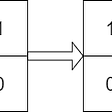# Fibonacci Iterative vs. Recursive

## Time Complexity:

`fib(n):    if n <= 1        return 1    return fib(n - 1) + fib(n - 2)`
`T(n) = T(n-1) + T(n-2) + c     = 2T(n-2) + c    //from the approximation T(n-1) ~ T(n-2)     = 2*(2T(n-4) + c) + c     = 4T(n-4) + 3c     = 8T(n-6) + 7c     = 2^k * T(n - 2k) + (2^k - 1)*cLet's find the value of k for which: n - 2k = 0k = n/2T(n) = 2^(n/2) * T(0) + (2^(n/2) - 1)*c     = 2^(n/2) * (1 + c) - ci.e. T(n) ~ 2^(n/2)`
`T(n) = T(n-1) + T(n-2) + c     = 2T(n-1) + c    //from the approximation T(n-1) ~ T(n-2)     = 2*(2T(n-2) + c) + c     = 4T(n-2) + 3c     = 8T(n-3) + 7c     = 2^k * T(n - k) + (2^k - 1)*cLet's find the value of k for which: n - k = 0k = nT(n) = 2^n * T(0) + (2^n - 1)*c     = 2^n * (1 + c) - ci.e. T(n) ~ 2^n`

--

--

--

## More from Syed Tousif Ahmed

Re-learning the fundamentals of programming.

Love podcasts or audiobooks? Learn on the go with our new app.

## Salesforce Data Security## DRY — Don’t Repeat Yourself## Dropped out of college to pursue programming## Create a YAML Manifest Template in Monokle## Code Stories: An Introduction## How to get a last-minute tech internship (college and pre-college)## Feature Engineering Part -1 End of Tail Imputation.## Syed Tousif Ahmed

Re-learning the fundamentals of programming.

## Helix Search ~ Searching Algorithm## BackTracking(1) / Algorithm## Leetcode 1886. Determine Whether Matrix Can Be Obtained By Rotation## Backtracking Algorithm